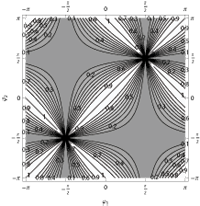## Stabilizing Non-Hermitian Systems by Periodic Driving

Jiangbin Gong, Qing-hai Wang

The time evolution of a system with a time-dependent non-Hermitian Hamiltonian is in general unstable with exponential growth or decay. A periodic driving field may stabilize the dynamics because the eigenphases of the associated Floquet operator may become all real. This possibility can emerge for a continuous range of system parameters with subtle domain boundaries. It is further shown that the issue of stability of a driven non-Hermitian Rabi model can be mapped onto the band structure problem of a class of lattice Hamiltonians. As an application, we show how to use the stability of driven non-Hermitian two-level systems (0-dimension in space) to simulate a spectrum analogous to Hofstadter’s butterfly that has played a paradigmatic role in quantum Hall physics. The simulation of the band structure of non-Hermitian superlattice potentials with parity-time reversal symmetry is also briefly discussed.

http://arxiv.org/abs/1412.3549
Quantum Physics (quant-ph)

## General PT-Symmetric Matrices

Jia-wen Deng, Uwe Guenther, Qing-hai Wang

Three ways of constructing a non-Hermitian matrix with possible all real eigenvalues are discussed. They are PT symmetry, pseudo-Hermiticity, and generalized PT symmetry. Parameter counting is provided for each class. All three classes of matrices have more real parameters than a Hermitian matrix with the same dimension. The generalized PT-symmetric matrices are most general among the three. All self-adjoint matrices process a generalized PT symmetry. For a given matrix, it can be both PT-symmetric and P’-pseudo-Hermitian with respect to some P’ operators. The relation between corresponding P and P’ operators is established. The Jordan block structures of each class are discussed. Explicit examples in 2×2 are shown.

http://arxiv.org/abs/1212.1861
Quantum Physics (quant-ph); Mathematical Physics (math-ph)

## Time Dependent PT-Symmetric Quantum Mechanics

Jiangbin Gong, Qing-hai Wang

The so-called parity-time-reversal- (PT-) symmetric quantum mechanics (PTQM) has developed into a noteworthy area of research. However, to date most known studies of PTQM focused on the spectral properties of non-Hermitian Hamiltonian operators. In this work, we propose an axiom in PTQM in order to study general time-dependent problems in PTQM, e.g., those with a time-dependent PT-symmetric Hamiltonian and with a time-dependent metric. We illuminate our proposal by examining a proper mapping from a time-dependent Schroedinger-like equation of motion for PTQM to the familiar time-dependent Schroedinger equation in conventional quantum mechanics. The rich structure of the proper mapping hints that time-dependent PTQM can be a fruitful extension of conventional quantum mechanics. Under our proposed framework, we further study in detail the Berry phase generation in a class of PT-symmetric two-level systems. It is found that a closed adiabatic path in PTQM is often associated with an open adiabatic path in a properly mapped problem in conventional quantum mechanics. In one interesting case we further interpret the Berry phase as the flux of a continuously tunable fictitious magnetic monopole, thus highlighting the difference between PTQM and conventional quantum mechanics despite the existence of a proper mapping between them.

http://arxiv.org/abs/1210.5344
Quantum Physics (quant-ph)

## 2*2 random matrix ensembles with reduced symmetry: From Hermitian to PT-symmetric matrices

Jiangbin Gong, Qing-hai Wang

A possibly fruitful extension of conventional random matrix ensembles is proposed by imposing symmetry constraints on conventional Hermitian matrices or parity-time- (PT-) symmetric matrices. To illustrate the main idea, we first study 2*2 complex Hermitian matrix ensembles with O(2) invariant constraints, yielding novel level-spacing statistics such as singular distributions, half-Gaussian distribution, distributions interpolating between GOE (Gaussian Orthogonal Ensemble) distribution and half Gaussian distributions, as well as gapped-GOE distribution. Such a symmetry-reduction strategy is then used to explore 2*2 PT-symmetric matrix ensembles with real eigenvalues. In particular, PT-symmetric random matrix ensembles with U(2) invariance can be constructed, with the conventional complex Hermitian random matrix ensemble being a special case. In two examples of PT-symmetric random matrix ensembles, the level-spacing distributions are found to be the standard GUE (Gaussian Unitary Ensemble) statistics or “truncated-GUE” statistics.

http://arxiv.org/abs/1204.6126
Quantum Physics (quant-ph)

## Entanglement Efficiencies in PT-Symmetric Quantum Mechanics

Christian Zielinski, Qing-hai WangThe degree of entanglement is determined for arbitrary states of a PT-symmetric bipartite composite system. We characterize the rate with which entangled states are generated and show that this rate can be quantified by a small set of parameters. These relations allow one in principle to increase the efficiency of these systems to entangle states. It is also noticed that many relations resemble corresponding ones in conventional quantum mechanics.

http://arxiv.org/abs/1106.3856
High Energy Physics – Theory (hep-th); Quantum Physics (quant-ph)